### Majestic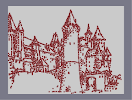Hover over the thumbnail for a full-size version.

Author mrgy05 art author:mrgy05 bitesized construction majestic mines n-art nart nonplayable rated 2007-11-15 2007-11-16 5 by 80 people. \$Majestic#mrgy05#none#11111111111111111111111P000000000000000000000NP000000000000000000000NP000000000000000000000NP000000000000000000000NP000000000000000000000NP000000000000000000000NP000000000000000000000NP000000000000000000000NP000000000000000000000NP000000000000000000000NP000000000000000000000NP000000000000000000000NP000000000000000000000NP000000000000000000000NP000000000000000000000NP000000000000000000000NP000000000000000000000NP000000000000000000000NP000000000000000000000NP000000000000000000000NP000000000000000000000NP000000000000000000000NP000000000000000000000NP000000000000000000000NP000000000000000000000NP000000000000000000000NP000000000000000000000NP000000000000000000000NP000000000000000000000N11111111111111111111111|12^490,526!12^489,521!12^487,512!12^485,504!12^484,499!12^484,491!12^482,485!12^482,480!12^481,475!12^480,468!12^480,463!12^480,456!12^480,448!12^478,442!12^479,434!12^476,424!12^476,418!12^476,409!12^476,401!12^476,394!12^475,390!12^475,383!12^475,379!12^470,377!12^462,374!12^425,369!12^418,370!12^383,378!12^387,376!12^410,370!12^447,370!12^440,370!12^433,370!12^455,371!12^402,372!12^396,373!12^379,381!12^380,373!12^379,364!12^381,354!12^381,344!12^382,334!12^381,327!12^382,321!12^382,310!12^382,302!12^382,293!12^382,288!12^381,277!12^381,268!12^382,259!12^382,252!12^382,245!12^381,236!12^382,233!12^382,225!12^382,219!12^382,211!12^381,203!12^381,196!12^381,192!12^377,188!12^391,184!12^395,181!12^401,180!12^405,180!12^422,180!12^422,178!12^419,181!12^439,181!12^438,179!12^438,183!12^455,184!12^455,181!12^468,185!12^466,187!12^462,191!12^391,192!12^388,197!12^389,206!12^394,195!12^403,188!12^395,187!12^386,194!12^389,213!12^401,205!12^398,202!12^407,198!12^409,187!12^420,190!12^420,186!12^431,188!12^421,198!12^411,208!12^398,220!12^387,226!12^389,241!12^393,231!12^408,234!12^389,367!12^389,274!12^390,255!12^473,370!12^473,360!12^472,351!12^471,343!12^471,335!12^472,326!12^471,317!12^471,311!12^470,303!12^470,296!12^469,286!12^469,280!12^469,270!12^470,264!12^469,254!12^469,247!12^469,240!12^469,232!12^469,224!12^469,215!12^471,207!12^471,197!12^470,189!12^474,186!12^477,184!12^478,176!12^479,170!12^477,162!12^474,161!12^470,160!12^467,171!12^467,166!12^465,167!12^462,163!12^457,158!12^447,163!12^448,155!12^443,156!12^439,159!12^437,156!12^435,151!12^413,153!12^407,156!12^408,160!12^405,160!12^401,152!12^396,152!12^387,155!12^387,164!12^387,158!12^394,166!12^397,170!12^390,173!12^384,179!12^379,174!12^375,182!12^373,172!12^373,167!12^379,159!12^384,152!12^379,158!12^388,150!12^389,145!12^391,139!12^395,134!12^398,127!12^402,120!12^405,114!12^405,109!12^408,102!12^400,118!12^398,126!12^393,135!12^413,96!12^417,88!12^420,80!12^423,71!12^423,63!12^421,130!12^399,139!12^392,147!12^403,146!12^417,141!12^424,139!12^436,140!12^470,156!12^466,153!12^458,149!12^453,146!12^442,144!12^434,143!12^424,143!12^417,143!12^408,143!12^454,151!12^443,151!12^434,150!12^425,150!12^418,149!12^412,149!12^409,149!12^436,145!12^451,148!12^406,137!12^414,132!12^406,128!12^405,126!12^410,115!12^409,109!12^413,104!12^417,100!12^410,129!12^421,119!12^420,109!12^420,95!12^411,121!12^466,150!12^460,144!12^454,136!12^449,128!12^446,121!12^443,113!12^442,104!12^439,97!12^436,91!12^433,86!12^431,79!12^430,75!12^429,69!12^426,65!12^424,60!12^417,168!12^429,168!12^441,168!12^451,170!12^462,174!12^470,177!12^405,170!12^391,173!12^380,175!12^359,546!12^360,538!12^361,530!12^364,522!12^365,514!12^366,505!12^368,498!12^369,489!12^369,480!12^371,474!12^372,464!12^373,457!12^373,450!12^375,440!12^375,431!12^377,422!12^377,411!12^377,404!12^377,396!12^378,389!12^380,386!12^401,548!12^401,541!12^401,534!12^404,530!12^409,527!12^411,528!12^419,536!12^421,546!12^420,523!12^413,517!12^398,524!12^396,537!12^396,546!12^415,439!12^414,440!12^411,440!12^411,435!12^413,433!12^413,437!12^454,553!12^449,553!12^439,557!12^430,559!12^385,550!12^366,550!12^357,549!12^350,549!12^339,549!12^330,549!12^322,549!12^315,550!12^498,548!12^493,540!12^487,550!12^480,551!12^493,552!12^495,558!12^499,553!12^508,553!12^521,539!12^520,531!12^518,523!12^518,514!12^517,507!12^516,495!12^517,488!12^517,480!12^515,471!12^519,468!12^525,465!12^529,463!12^535,462!12^545,462!12^551,462!12^558,462!12^564,463!12^569,468!12^573,470!12^579,539!12^576,530!12^575,522!12^575,514!12^575,507!12^573,501!12^573,495!12^573,489!12^573,482!12^573,475!12^527,540!12^531,537!12^534,535!12^533,526!12^533,519!12^532,512!12^532,507!12^531,503!12^529,497!12^529,492!12^528,486!12^527,479!12^528,475!12^530,474!12^532,470!12^535,465!12^490,487!12^489,476!12^489,468!12^489,458!12^487,452!12^493,451!12^494,462!12^495,469!12^496,455!12^506,456!12^504,459!12^504,451!12^516,452!12^525,448!12^494,493!12^502,478!12^506,468!12^531,448!12^535,448!12^546,447!12^551,447!12^555,447!12^559,447!12^565,445!12^571,445!12^576,443!12^581,443!12^589,442!12^595,441!12^600,441!12^605,440!12^612,445!12^614,442!12^623,442!12^626,441!12^630,441!12^645,440!12^642,437!12^634,435!12^625,433!12^645,455!12^645,448!12^651,444!12^654,441!12^655,435!12^659,433!12^664,431!12^665,430!12^657,427!12^651,430!12^646,425!12^642,423!12^639,422!12^633,425!12^628,426!12^622,427!12^616,430!12^609,433!12^603,432!12^598,428!12^594,430!12^592,428!12^590,422!12^584,422!12^580,424!12^575,420!12^569,427!12^564,429!12^562,433!12^558,436!12^552,435!12^548,438!12^546,441!12^659,448!12^669,447!12^662,452!12^662,457!12^654,458!12^650,457!12^650,467!12^652,453!12^656,451!12^644,430!12^632,429!12^593,434!12^583,430!12^580,435!12^576,436!12^568,436!12^561,437!12^580,429!12^594,434!12^365,495!12^359,489!12^359,484!12^361,480!12^357,476!12^353,472!12^349,467!12^349,473!12^348,472!12^348,463!12^348,459!12^345,455!12^342,455!12^336,461!12^335,468!12^332,465!12^329,455!12^327,451!12^329,446!12^327,442!12^320,443!12^314,446!12^312,438!12^311,432!12^306,429!12^305,437!12^296,438!12^292,432!12^287,430!12^283,437!12^276,432!12^376,389!12^371,389!12^369,394!12^363,398!12^357,399!12^354,410!12^352,408!12^347,410!12^341,412!12^334,414!12^329,417!12^324,417!12^320,420!12^315,421!12^310,421!12^305,423!12^301,426!12^292,427!12^289,429!12^282,429!12^295,428!12^338,449!12^336,440!12^326,431!12^323,429!12^365,467!12^365,471!12^352,457!12^363,459!12^355,463!12^358,456!12^352,448!12^342,444!12^341,429!12^333,432!12^335,426!12^342,424!12^355,445!12^358,432!12^349,437!12^352,440!12^369,452!12^365,445!12^374,423!12^367,433!12^362,435!12^363,424!12^366,412!12^369,407!12^355,427!12^353,426!12^355,416!12^338,420!12^323,430!12^384,391!12^388,386!12^387,406!12^385,418!12^394,393!12^403,379!12^420,381!12^404,395!12^425,215!12^440,196!12^571,532!12^567,532!12^561,532!12^567,526!12^563,522!12^554,519!12^556,515!12^560,508!12^562,503!12^563,497!12^562,491!12^565,489!12^565,484!12^565,487!12^567,515!12^567,509!12^565,500!12^587,541!12^592,541!12^599,541!12^610,541!12^519,540!12^515,540!12^508,540!12^630,540!12^628,533!12^630,529!12^631,525!12^632,521!12^678,519!12^673,519!12^665,519!12^658,517!12^651,517!12^645,517!12^639,516!12^637,514!12^642,513!12^647,508!12^650,505!12^686,509!12^680,508!12^672,508!12^667,507!12^662,507!12^656,503!12^631,515!12^631,511!12^629,502!12^629,495!12^630,488!12^630,480!12^631,474!12^630,469!12^630,464!12^630,457!12^630,450!12^642,453!12^638,448!12^639,441!12^614,456!12^614,459!12^615,462!12^616,460!12^616,457!12^599,460!12^598,462!12^599,463!12^601,460!12^600,457!12^599,458!12^634,459!12^651,500!12^651,496!12^650,492!12^645,491!12^644,491!12^644,479!12^644,471!12^643,464!12^723,451!12^719,448!12^715,450!12^710,454!12^705,459!12^699,462!12^697,457!12^695,457!12^695,461!12^690,455!12^687,455!12^679,455!12^678,459!12^678,465!12^672,466!12^666,471!12^701,496!12^697,494!12^694,497!12^691,494!12^689,488!12^689,482!12^685,494!12^681,496!12^675,493!12^674,488!12^672,483!12^670,491!12^664,488!12^658,483!12^658,484!12^666,476!12^656,471!12^656,479!12^656,488!12^667,496!12^661,529!12^661,531!12^659,531!12^660,528!12^679,533!12^676,533!12^678,529!12^643,532!12^646,532!12^644,528!12^637,545!12^647,545!12^663,545!12^631,545!12^318,531!12^343,520!12^347,515!12^272,432!12^261,432!12^252,431!12^244,428!12^237,428!12^225,430!12^219,429!12^212,432!12^219,431!12^219,424!12^218,417!12^218,409!12^217,401!12^217,396!12^255,392!12^251,392!12^245,392!12^240,393!12^234,393!12^226,393!12^221,395!12^289,398!12^284,397!12^276,396!12^273,395!12^265,393!12^264,393!12^255,387!12^254,383!12^254,375!12^253,373!12^252,369!12^251,366!12^249,361!12^247,356!12^246,353!12^218,391!12^221,384!12^224,379!12^226,375!12^231,371!12^236,365!12^236,360!12^242,357!12^229,386!12^233,380!12^235,375!12^239,370!12^239,384!12^242,376!12^244,370!12^248,385!12^248,378!12^290,394!12^287,390!12^282,384!12^279,379!12^273,374!12^269,369!12^264,363!12^262,359!12^259,355!12^255,352!12^254,351!12^261,427!12^260,427!12^260,420!12^260,413!12^255,403!12^259,400!12^258,406!12^243,422!12^234,423!12^231,425!12^230,416!12^230,407!12^239,419!12^235,408!12^236,401!12^248,417!12^245,406!12^246,401!12^222,421!12^226,404!12^274,425!12^273,420!12^274,413!12^274,410!12^277,416!12^277,420!12^277,424!12^289,422!12^289,418!12^288,409!12^289,403!12^336,411!12^336,407!12^336,403!12^337,396!12^337,386!12^336,378!12^335,372!12^335,366!12^334,357!12^335,348!12^336,344!12^338,342!12^345,339!12^350,339!12^356,397!12^356,388!12^355,381!12^355,374!12^354,366!12^355,360!12^355,353!12^354,346!12^354,345!12^348,398!12^343,399!12^339,403!12^344,402!12^348,397!12^350,392!12^340,395!12^342,392!12^346,389!12^349,386!12^350,383!12^338,385!12^341,381!12^343,379!12^346,377!12^344,375!12^340,377!12^343,371!12^345,370!12^349,367!12^349,362!12^339,364!12^342,363!12^344,360!12^346,356!12^338,356!12^340,354!12^342,351!12^345,349!12^185,426!12^185,422!12^184,418!12^184,412!12^184,399!12^185,395!12^185,388!12^182,386!12^180,382!12^149,426!12^149,420!12^149,413!12^145,404!12^146,395!12^146,386!12^144,381!12^142,377!12^145,425!12^143,426!12^141,427!12^142,422!12^142,416!12^141,408!12^140,399!12^139,391!12^139,384!12^139,380!12^164,371!12^158,367!12^150,369!12^150,372!12^155,371!12^202,425!12^204,424!12^204,415!12^203,408!12^201,399!12^200,390!12^197,384!12^194,379!12^208,418!12^209,412!12^209,401!12^208,395!12^205,384!12^210,379!12^204,379!12^202,377!12^83,401!12^82,397!12^81,394!12^81,383!12^80,369!12^80,364!12^81,361!12^132,366!12^127,363!12^121,361!12^116,360!12^108,360!12^102,360!12^96,358!12^85,356!12^80,355!12^73,358!12^63,359!12^53,362!12^48,362!12^92,335!12^89,338!12^83,343!12^76,344!12^71,346!12^61,352!12^57,353!12^62,351!12^72,346!12^85,340!12^87,337!12^83,353!12^85,350!12^85,345!12^88,342!12^73,348!12^70,351!12^75,354!12^75,402!12^71,390!12^70,382!12^70,373!12^71,370!12^62,377!12^63,388!12^63,392!12^57,375!12^63,373!12^61,408!12^100,364!12^101,367!12^98,372!12^95,374!12^95,370!12^95,365!12^119,369!12^120,370!12^117,377!12^116,375!12^117,371!12^118,368!12^134,418!12^130,416!12^122,416!12^114,415!12^106,415!12^101,415!12^138,431!12^131,427!12^120,428!12^114,428!12^127,432!12^123,430!12^130,430!12^138,428!12^167,430!12^164,431!12^156,430!12^148,430!12^196,374!12^192,373!12^185,373!12^179,372!12^172,372!12^200,371!12^199,368!12^194,362!12^193,358!12^189,355!12^182,351!12^174,351!12^167,350!12^161,350!12^153,349!12^188,348!12^190,344!12^188,338!12^190,326!12^187,323!12^185,319!12^185,332!12^179,331!12^171,330!12^167,327!12^159,326!12^152,326!12^181,316!12^177,316!12^169,314!12^160,311!12^193,325!12^203,321!12^211,319!12^220,316!12^227,365!12^225,357!12^226,351!12^226,345!12^226,333!12^226,327!12^226,322!12^242,349!12^242,343!12^239,335!12^238,328!12^238,320!12^237,314!12^237,309!12^234,304!12^220,309!12^220,305!12^218,302!12^216,299!12^191,324!12^197,322!12^202,315!12^201,310!12^205,308!12^207,304!12^198,316!12^196,316!12^210,299!12^211,309!12^202,360!12^200,354!12^199,345!12^199,337!12^197,330!12^211,371!12^210,365!12^208,353!12^207,344!12^207,335!12^219,366!12^218,359!12^215,351!12^217,378!12^214,378!12^213,371!12^214,362!12^197,350!12^153,333!12^152,340!12^157,336!12^160,339!12^164,336!12^170,337!12^174,340!12^112,335!12^110,328!12^112,321!12^111,313!12^111,310!12^121,309!12^117,308!12^112,305!12^105,311!12^103,311!12^100,315!12^100,314!12^105,309!12^107,308!12^106,333!12^104,324!12^121,318!12^132,361!12^126,356!12^121,351!12^117,348!12^109,341!12^104,339!12^97,335!12^126,342!12^126,336!12^126,328!12^126,319!12^126,316!12^143,360!12^139,356!12^128,347!12^143,371!12^146,350!12^142,351!12^141,353!12^89,182!12^86,181!12^82,181!12^78,179!12^73,176!12^62,175!12^59,175!12^60,175!12^63,173!12^70,173!12^68,168!12^69,165!12^67,158!12^67,152!12^66,146!12^66,139!12^63,132!12^62,127!12^62,119!12^61,111!12^61,102!12^61,97!12^58,88!12^58,82!12^58,79!12^97,169!12^92,163!12^87,152!12^86,144!12^81,137!12^78,127!12^75,121!12^68,111!12^71,108!12^65,99!12^65,92!12^59,165!12^52,167!12^51,163!12^55,157!12^55,155!12^54,146!12^52,140!12^57,132!12^55,125!12^52,117!12^53,176!12^65,178!12^64,181!12^63,186!12^63,191!12^58,185!12^56,184!12^52,183!12^88,192!12^86,190!12^83,189!12^75,186!12^71,186!12^87,209!12^84,211!12^79,214!12^80,220!12^61,207!12^62,205!12^62,211!12^65,214!12^72,338!12^69,330!12^69,323!12^70,315!12^70,307!12^70,296!12^71,287!12^71,281!12^71,273!12^71,265!12^71,258!12^70,251!12^70,242!12^70,235!12^69,227!12^69,220!12^69,216!12^85,333!12^85,328!12^84,318!12^82,332!12^82,320!12^82,313!12^82,305!12^82,294!12^70,292!12^83,288!12^83,281!12^82,285!12^83,274!12^81,263!12^81,256!12^80,252!12^81,242!12^80,235!12^81,229!12^81,225!12^91,231!12^91,224!12^91,216!12^95,213!12^97,210!12^95,202!12^72,205!12^49,206!12^49,209!12^55,231!12^55,235!12^55,240!12^113,450!12^110,444!12^108,437!12^102,435!12^111,434!12^106,429!12^105,426!12^103,421!12^98,416!12^91,415!12^89,411!12^86,407!12^80,406!12^79,410!12^70,408!12^65,410!12^99,435!12^97,441!12^89,445!12^90,452!12^86,456!12^85,459!12^83,464!12^82,470!12^82,479!12^82,483!12^82,488!12^80,492!12^74,501!12^72,484!12^76,481!12^74,471!12^73,465!12^77,463!12^77,460!12^76,455!12^79,452!12^83,442!12^82,439!12^88,436!12^90,434!12^93,432!12^93,428!12^93,422!12^95,427!12^99,427!12^97,425!12^90,424!12^85,430!12^79,433!12^75,432!12^75,426!12^68,428!12^87,440!12^80,442!12^75,444!12^74,440!12^67,441!12^64,445!12^61,444!12^55,442!12^55,449!12^74,460!12^71,461!12^66,463!12^61,465!12^61,470!12^69,517!12^65,522!12^65,527!12^702,472!12^704,466!12^711,466!12^719,470!12^721,465!12^726,475!12^726,476!12^714,473!12^711,469!12^704,472!12^702,471!12^692,465!12^200,430!12^194,430!12^184,431!12^147,385!12^151,385!12^154,384!12^217,290!12^217,284!12^217,279!12^217,273!12^216,271!12^214,270!12^209,269!12^203,268!12^198,268!12^193,271!12^187,274!12^184,277!12^182,278!12^187,306!12^187,300!12^188,294!12^182,301!12^182,297!12^178,292!12^176,290!12^172,290!12^165,289!12^160,286!12^155,284!12^148,283!12^144,283!12^149,285!12^169,290!12^168,294!12^163,301!12^163,301!12^147,305!12^145,301!12^146,293!12^145,288!12^127,305!12^129,299!12^131,293!12^131,287!12^130,279!12^130,272!12^130,266!12^130,261!12^130,257!12^129,251!12^129,244!12^128,234!12^129,222!12^128,211!12^128,207!12^127,198!12^127,193!12^122,192!12^116,191!12^113,191!12^103,196!12^101,200!12^108,300!12^112,292!12^112,283!12^112,272!12^112,260!12^112,252!12^112,244!12^112,236!12^109,225!12^112,224!12^112,217!12^112,208!12^111,200!12^104,229!12^100,260!12^95,308!12^95,301!12^96,295!12^94,282!12^94,263!12^94,257!12^94,248!12^94,242!12^94,233!12^101,287!12^101,281!12^104,270!12^97,274!12^103,256!12^101,252!12^100,246!12^102,241!12^101,227!12^102,218!12^101,210!12^88,323!12^89,317!12^90,311!12^90,308!12^90,297!12^90,290!12^90,285!12^89,279!12^88,275!12^90,267!12^87,255!12^87,249!12^86,241!12^87,234!12^86,224!12^87,265!12^87,272!12^125,184!12^126,181!12^123,183!12^120,185!12^118,181!12^113,181!12^112,181!12^126,182!12^102,187!12^105,183!12^109,177!12^110,171!12^109,167!12^111,160!12^112,155!12^113,149!12^114,147!12^115,142!12^118,137!12^119,128!12^108,166!12^102,172!12^99,178!12^107,164!12^106,177!12^96,187!12^97,192!12^96,193!12^93,192!12^95,183!12^92,183!12^141,188!12^138,184!12^133,182!12^141,181!12^53,90!12^56,85!12^48,100!12^128,154!12^126,145!12^123,135!12^120,125!12^135,172!12^139,197!12^134,197!12^133,195!12^142,225!12^142,221!12^140,212!12^141,204!12^142,277!12^139,271!12^140,263!12^140,256!12^140,243!12^140,234!12^175,277!12^170,277!12^166,275!12^158,273!12^154,273!12^147,269!12^179,270!12^182,266!12^187,264!12^193,264!12^194,262!12^188,268!12^192,258!12^196,256!12^193,252!12^201,260!12^200,247!12^201,242!12^203,237!12^206,230!12^207,226!12^207,218!12^208,260!12^205,254!12^207,246!12^214,263!12^213,261!12^222,265!12^218,260!12^213,250!12^210,244!12^209,237!12^238,270!12^233,270!12^228,267!12^235,277!12^229,273!12^222,270!12^244,277!12^230,264!12^226,258!12^227,252!12^223,248!12^220,245!12^220,241!12^218,236!12^213,230!12^212,222!12^233,282!12^233,290!12^233,297!12^193,241!12^186,238!12^179,236!12^170,233!12^164,229!12^158,227!12^153,224!12^149,223!12^144,222!12^67,340!12^62,340!12^57,340!12^53,339!12^343,326!12^333,324!12^325,323!12^320,323!12^309,320!12^300,317!12^290,316!12^283,315!12^274,312!12^268,311!12^255,306!12^250,306!12^244,305!12^239,304!12^261,308!12^267,343!12^267,340!12^267,348!12^256,338!12^306,349!12^305,351!12^315,352!12^315,358!12^313,362!12^324,351!12^324,357!12^312,329!12^303,329!12^304,326!12^266,317!12^269,319!12^257,316!12^325,405!12^325,403!12^325,401!12^324,396!12^304,371!12^421,257!12^419,258!12^421,262!12^421,266!12^445,258!12^445,262!12^408,545!12^408,544!12^409,540!12^410,538!12^410,542!12^410,547!12^407,545!12^402,546!12^402,539!12^402,534!12^191,401!12^192,402!12^190,408!12^194,412!12^169,390!12^106,410!12^125,412!12^297,299!12^295,297!12^295,293!12^294,287!12^292,285!12^283,298!12^287,294!12^288,289!12^290,285!12^280,294!12^280,289!12^280,283!12^280,278!12^280,271!12^280,263!12^280,262!12^279,262!12^293,282!12^292,275!12^292,269!12^280,288!12^276,290!12^274,286!12^269,281!12^275,280!12^272,280!12^259,297!12^262,297!12^248,294!12^286,303!12^296,304!12^321,308!12^315,303!12^314,300!12^308,296!12^304,291!12^319,298!12^318,299!12^322,307!12^322,297!12^321,289!12^321,282!12^321,274!12^319,265!12^317,281!12^312,280!12^307,279!12^303,276!12^301,275!12^301,271!12^312,275!12^309,272!12^302,265!12^305,259!12^307,253!12^310,249!12^312,243!12^315,237!12^318,231!12^320,222!12^320,216!12^323,211!12^324,203!12^318,228!12^315,235!12^312,245!12^310,265!12^313,258!12^317,248!12^316,269!12^322,262!12^323,258!12^323,253!12^326,249!12^327,244!12^330,236!12^331,232!12^331,228!12^333,220!12^334,214!12^334,209!12^335,204!12^335,195!12^329,254!12^339,249!12^334,252!12^342,252!12^342,252!12^339,252!12^356,259!12^353,258!12^349,256!12^346,255!12^355,303!12^357,323!12^352,322!12^350,318!12^349,311!12^348,306!12^348,299!12^349,292!12^346,278!12^347,271!12^347,263!12^354,281!12^352,273!12^354,315!12^357,309!12^354,294!12^355,266!12^332,316!12^328,313!12^326,311!12^330,306!12^330,301!12^329,288!12^328,282!12^329,273!12^332,266!12^330,261!12^342,256!12^338,254!12^335,255!12^344,246!12^344,239!12^355,253!12^351,248!12^347,237!12^347,232!12^342,215!12^363,269!12^367,266!12^367,266!12^365,263!12^365,260!12^367,256!12^368,253!12^367,248!12^368,243!12^370,237!12^372,233!12^378,250!12^376,246!12^376,269!12^371,271!12^368,273!12^361,274!12^361,276!12^339,287!12^339,284!12^340,314!12^340,316!12^274,270!12^267,269!12^262,266!12^256,265!12^251,262!12^244,260!12^238,257!12^231,254!12^285,274!12^366,338!12^366,334!12^366,327!12^372,329!12^372,322!12^375,318!12^377,314!12^377,313!12^372,317!12^366,313!12^363,308!12^365,305!12^366,297!12^368,291!12^369,285!12^372,281!12^373,276!12^380,295!12^378,289!12^377,283!12^372,299!12^370,301!12^371,297!12^362,330!12^359,335!12^356,330!12^366,324!12^360,316!12^375,338!12^369,387!12^369,384!12^369,378!12^369,371!12^372,378!12^372,374!12^369,366!12^371,360!12^371,354!12^368,346!12^368,355!12^373,386!12^350,405!12^344,405!12^339,406!12^276,343!12^276,343!12^277,316!12^534,435!12^531,430!12^527,424!12^526,421!12^521,415!12^530,437!12^525,437!12^521,437!12^517,437!12^514,437!12^514,430!12^516,426!12^517,420!12^512,440!12^512,448!12^510,444!12^508,442!12^538,442!12^523,445!12^520,434!12^506,488!12^498,482!12^494,478!12^501,472!12^494,507!12^494,500!12^494,493!12^492,487!12^488,470!12^486,465!12^484,462!12^485,458!12^498,468!12^499,461!12^517,462!12^512,457!12^509,480!12^504,493!12^501,507!12^525,506!12^525,518!12^523,524!12^524,528!12^526,511!12^522,508!12^522,498!12^522,493!12^521,487!12^522,479!12^523,472!12^526,466!12^535,463!12^539,431!12^495,447!12^488,447!12^588,411!12^583,406!12^583,402!12^581,398!12^579,394!12^579,390!12^577,388!12^577,386!12^575,383!12^575,378!12^575,375!12^572,371!12^596,411!12^598,409!12^602,405!12^606,401!12^608,394!12^608,388!12^609,383!12^608,376!12^607,368!12^590,401!12^590,401!12^594,401!12^595,391!12^591,391!12^587,390!12^590,379!12^588,372!12^583,374!12^579,374!12^587,374!12^589,374!12^579,373!12^577,368!12^577,363!12^609,361!12^602,361!12^594,360!12^589,360!12^583,361!12^576,362!12^570,363!12^570,347!12^580,353!12^573,356!12^572,358!12^570,340!12^572,333!12^578,343!12^582,351!12^590,356!12^589,348!12^577,331!12^598,375!12^584,388!12^620,388!12^619,378!12^619,370!12^615,385!12^615,389!12^620,371!12^618,367!12^613,369!12^618,369!12^623,367!12^617,359!12^616,353!12^615,346!12^615,340!12^612,334!12^615,331!12^619,331!12^623,331!12^622,339!12^621,347!12^620,354!12^621,359!12^621,350!12^626,401!12^626,398!12^622,390!12^625,388!12^625,383!12^626,380!12^625,375!12^625,371!12^626,359!12^626,355!12^626,349!12^626,345!12^626,338!12^637,354!12^638,352!12^633,336!12^637,370!12^652,362!12^651,364!12^646,364!12^639,362!12^632,362!12^647,359!12^646,355!12^646,350!12^647,344!12^646,341!12^645,338!12^646,331!12^648,335!12^650,398!12^649,390!12^649,386!12^649,382!12^647,377!12^647,372!12^651,378!12^652,378!12^651,390!12^652,389!12^655,410!12^653,406!12^659,403!12^661,393!12^659,381!12^662,378!12^661,371!12^664,367!12^663,364!12^659,364!12^654,364!12^665,360!12^664,352!12^664,332!12^661,331!12^656,330!12^651,330!12^667,330!12^664,326!12^662,323!12^660,319!12^656,315!12^654,310!12^652,306!12^650,301!12^647,296!12^644,292!12^644,288!12^642,284!12^643,323!12^641,318!12^637,311!12^635,307!12^633,304!12^634,299!12^637,297!12^639,293!12^642,290!12^642,312!12^641,309!12^639,302!12^640,297!12^652,336!12^652,340!12^657,427!12^657,423!12^657,417!12^663,426!12^667,426!12^669,429!12^664,435!12^663,439!12^663,441!12^679,475!12^677,477!12^677,481!12^680,474!12^700,508!12^694,500!12^692,504!12^684,506!12^676,502!12^671,501!12^668,503!12^663,499!12^658,495!12^656,496!12^663,495!12^675,501!12^683,498!12^684,500!12^688,502!12^693,503!12^700,505!12^703,510!12^699,487!12^699,484!12^701,479!12^704,499!12^710,499!12^612,549!12^607,550!12^599,551!12^596,555!12^577,545!12^571,549!12^571,541!12^566,543!12^559,545!12^557,546!12^553,549!12^570,535!12^539,517!12^548,513!12^548,511!12^548,508!12^548,473!12^548,473!12^564,471!12^537,398!12^537,396!12^537,395!12^536,370!12^536,372!12^536,374!12^552,341!12^552,343!12^535,345!12^564,417!12^573,413!12^577,403!12^569,403!12^567,409!12^575,390!12^568,385!12^566,373!12^565,393!12^562,405!12^547,431!12^540,421!12^519,410!12^519,397!12^519,393!12^519,385!12^518,374!12^518,369!12^517,361!12^520,356!12^519,350!12^517,341!12^518,345!12^517,354!12^526,338!12^522,340!12^523,343!12^532,339!12^536,339!12^541,337!12^547,335!12^551,334!12^557,334!12^562,334!12^566,333!12^367,535!12^372,518!12^374,497!12^375,483!12^380,470!12^380,454!12^380,438!12^387,430!12^393,404!12^383,395!12^393,385!12^397,381!12^398,256!12^397,244!12^399,232!12^412,216!12^415,203!12^424,195!12^409,187!12^389,214!12^388,205!12^390,197!12^402,194!12^395,200!12^396,206!12^389,222!12^398,223!12^399,212!12^383,212!12^383,206!12^392,201!12^388,234!12^393,219!12^398,215!12^405,208!12^411,196!12^404,217!12^400,224!12^399,137!12^405,131!12^406,126!12^406,121!12^408,117!12^411,112!12^414,92!12^417,89!12^417,84!12^418,79!12^421,72!12^411,99!12^400,134!12^396,142!12^395,146!12^409,155!12^404,150!12^386,159!12^425,148!12^413,160!12^443,160!12^463,159!12^461,156!12^613,327!12^609,319!12^606,315!12^605,311!12^603,307!12^600,301!12^598,295!12^598,291!12^595,283!12^594,279!12^592,276!12^571,321!12^573,315!12^577,311!12^581,305!12^578,303!12^579,297!12^584,291!12^586,288!12^587,283!12^588,276!12^590,273!12^581,299!12^576,308!12^582,314!12^578,321!12^581,324!12^585,321!12^589,319!12^599,319!12^584,315!12^586,310!12^586,302!12^588,298!12^590,308!12^588,334!12^588,331!12^602,333!12^602,331!12^627,325!12^625,321!12^622,319!12^619,316!12^615,311!12^628,330!12^633,330!12^636,330!12^639,330!12^629,313!12^630,307!12^620,306!12^609,302!12^606,302!12^629,301!12^627,295!12^627,290!12^627,286!12^627,281!12^624,274!12^625,273!12^625,267!12^626,261!12^626,258!12^626,256!12^622,259!12^611,261!12^611,257!12^610,254!12^610,256!12^608,258!12^595,259!12^598,255!12^596,252!12^590,262!12^583,262!12^580,256!12^580,254!12^579,259!12^575,263!12^571,264!12^571,257!12^569,255!12^567,260!12^566,266!12^563,272!12^563,268!12^561,269!12^562,289!12^562,283!12^562,278!12^567,285!12^567,278!12^567,272!12^571,273!12^575,270!12^580,266!12^575,282!12^582,276!12^593,266!12^616,277!12^614,277!12^613,281!12^615,278!12^632,240!12^626,240!12^621,238!12^616,236!12^612,236!12^603,234!12^596,234!12^589,234!12^581,234!12^574,234!12^567,238!12^563,238!12^558,240!12^555,244!12^560,254!12^561,246!12^566,246!12^568,247!12^574,241!12^581,243!12^590,244!12^587,246!12^595,242!12^606,241!12^611,243!12^597,235!12^585,237!12^552,232!12^557,226!12^561,223!12^564,218!12^568,211!12^571,201!12^579,193!12^573,194!12^577,188!12^580,182!12^583,175!12^583,169!12^588,164!12^585,157!12^587,162!12^632,237!12^624,231!12^618,223!12^615,219!12^610,209!12^609,206!12^609,209!12^607,213!12^604,205!12^602,199!12^599,192!12^597,186!12^596,182!12^593,176!12^590,171!12^593,206!12^592,202!12^594,209!12^590,208!12^563,232!12^558,232!12^564,222!12^571,219!12^570,230!12^572,225!12^579,223!12^574,213!12^576,202!12^556,234!12^555,236!12^549,283!12^549,278!12^549,272!12^549,268!12^549,261!12^548,256!12^545,251!12^544,246!12^546,243!12^547,238!12^549,236!12^548,244!12^547,250!12^549,256!12^561,263!12^560,261!12^568,293!12^579,294!12^573,294!12^568,294!12^561,294!12^558,294!12^554,295!12^549,295!12^543,295!12^540,295!12^534,295!12^514,337!12^511,337!12^515,331!12^518,325!12^520,319!12^521,314!12^525,310!12^528,303!12^531,300!12^532,314!12^539,306!12^540,300!12^535,302!12^522,325!12^552,305!12^548,300!12^549,328!12^535,333!12^564,306!12^562,322!12^547,313!12^533,319!12^526,325!12^526,334!12^537,323!12^473,270!12^480,271!12^483,273!12^487,275!12^494,277!12^499,277!12^507,282!12^509,281!12^512,283!12^521,285!12^525,285!12^530,286!12^535,288!12^524,276!12^518,267!12^514,263!12^513,261!12^514,259!12^520,259!12^527,273!12^521,266!12^520,263!12^526,262!12^526,257!12^527,251!12^527,248!12^537,251!12^535,248!12^538,243!12^537,239!12^536,231!12^539,229!12^542,228!12^526,240!12^526,235!12^526,230!12^526,226!12^527,224!12^516,238!12^515,238!12^514,229!12^514,221!12^515,216!12^515,213!12^515,209!12^512,207!12^509,204!12^512,201!12^515,200!12^516,198!12^522,209!12^520,205!12^522,203!12^525,199!12^528,198!12^530,198!12^536,198!12^537,198!12^552,203!12^548,202!12^544,200!12^522,223!12^537,206!12^544,220!12^545,212!12^545,208!12^547,206!12^530,215!12^522,194!12^523,189!12^526,183!12^526,176!12^529,161!12^530,152!12^531,145!12^546,195!12^542,191!12^552,199!12^537,182!12^535,170!12^535,163!12^528,190!12^529,187!12^529,187!12^529,180!12^535,188!12^533,191!12^528,192!12^515,244!12^512,243!12^505,239!12^507,238!12^509,235!12^501,237!12^495,234!12^493,234!12^492,232!12^494,228!12^494,225!12^494,222!12^498,223!12^501,225!12^502,226!12^487,232!12^483,232!12^479,229!12^473,227!12^470,227!12^486,226!12^485,223!12^486,223!12^508,271!12^517,273!12^498,273!12^479,263!12^488,265!12^476,250!12^476,243!12^477,237!12^476,234!12^476,257!12^489,242!12^481,240!12^481,250!12^497,384!12^498,375!12^498,367!12^498,363!12^498,360!12^507,376!12^507,380!12^489,366!12^485,361!12^487,358!12^488,355!12^497,330!12^498,326!12^497,324!12^498,321!12^498,300!12^498,294!12^499,293!12^482,285!12^485,293!12^480,295!12^479,281!12^478,278!12^476,277!12^476,291!12^474,294!12^485,314!12^485,303!12^481,280!12^490,280!12^479,303!12^637,401!12^639,396!12^640,399!12^455,130!12^455,126!12^455,123!12^454,118!12^454,116!12^194,436!12^194,440!12^194,447!12^185,435!12^175,435!12^167,435!12^167,441!12^774,572!12^770,572!12^764,572!12^763,572!12^763,576!12^763,581!12^767,582!12^770,582!12^773,584!12^774,587!12^770,589!12^767,592!12^763,591!12^750,572!12^747,572!12^743,575!12^741,578!12^741,582!12^744,585!12^747,585!12^749,585!12^752,584!12^754,581!12^754,577!12^722,576!12^725,577!12^728,580!12^729,581!12^722,575!12^731,580!12^736,575!12^735,578!12^734,585!12^731,587!12^726,587!12^709,575!12^708,574!12^702,574!12^699,583!12^700,584!12^706,584!12^709,584!12^709,582!12^713,582!12^713,586!12^700,576!12^698,579!12^696,581!12^678,586!12^678,582!12^678,578!12^678,574!12^679,572!12^682,572!12^683,576!12^680,581!12^684,584!12^688,584!12^689,584!12^691,590!12^647,586!12^652,584!12^656,579!12^660,577!12^661,574!12^661,586!12^661,583!12^662,579!12^662,578!12^670,588!12^669,584!12^666,582!12^653,583!12^655,580!12^655,579!12^654,577!12^363,524!12^365,507!12^367,494!12^370,485!12^372,471!12^371,456!12^374,441!12^376,432!12^377,417!12^377,403!12^378,398!12^385,403!12^389,443!12^397,421!12^409,405!12^422,391!12^449,381!12^385,485!12^391,465!12^383,510!12^385,364!12^385,341!12^326,206!12^322,212!12^341,208!12^334,199!12^345,221!12^350,244!12^335,247!12^306,269!12^308,263!12^138,177!12^133,165!12^129,151!12^114,177!12^115,169!12^117,154!12^52,96!12^52,101!12^51,104!12^48,106!12^47,110!12^48,117!12^58,93!12^60,88!12^69,102!12^72,116!12^92,159!12^96,173!12^61,148!12^60,139!12^50,153!12^49,129!12^54,106!12^61,162!12^50,189!12^50,191!12^83,195!12^84,197!12^86,197!12^88,197!12^79,190!12^78,208!12^55,220!12^51,333!12^51,320!12^58,328!12^569,207!12^567,214!12^525,183!12^530,161!12^528,168!12^528,174!12^531,156!12^519,197!12^525,501!12^525,483!12^528,469!12^541,463!12^567,503!12^572,521!12^570,493!12^351,550!12^295,550!12^360,550!12^356,551!12^697,512!12^699,529!12^694,535!12^697,533!12^694,532!12^689,521!12^581,314!12^597,289!12^163,295!12^158,296!12^157,292!12^156,292!12^150,292!12^178,299!12^163,332!12^163,332!12^147,323!12^151,318!12^156,314!12^150,309!12^157,299!12^192,313!12^191,307!12^192,303!12^198,307!12^198,305!12^191,284!12^193,290!12^195,282!12^200,291!12^195,294!12^198,277!12^190,280!12^198,300!12^203,301!12^194,289!12^192,276!12^203,357!12^203,350!12^201,343!12^199,324!12^203,329!12^208,328!12^204,355!12^213,361!12^205,382!12^207,369!12^96,245!12^92,246!12^92,244!12^92,236!12^92,235!12^89,222!12^93,217!12^97,212!12^88,188!12^75,183!12^66,176!12^53,178!12^321,275!12^321,273!12^321,269!12^350,264!12^351,262!12^338,257!12^383,261!12^364,354!12^365,373!12^324,327!12^325,331!12^322,334!12^317,323!12^294,318!12^486,437!12^494,438!12^485,423!12^494,429!12^502,434!12^485,409!12^482,376!12^489,382!12^488,369!12^481,346!12^488,393!12^510,392!12^509,348!12^274,361!12^284,356# Well I was thinking of something that was new for a N-Art then I noticed that there wasn't one type of construction N-Art. What I was thinking making this N-Art: 'ugh, that's a huge castle' and 'ugh my wrist hurts' Music I listened (listed?) to making this N-Art: Modest Mouse, Dashboard, Matisyahu, and Kamelot. So, here it is! Majestic.

## Other maps by this author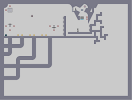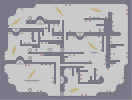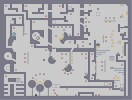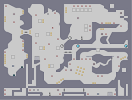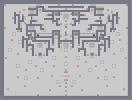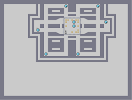Simply Difficult Don't fret precious I'm here... Follow That Drone! Head Hunter Fake Kill Count

Pages: (0)

### Woohoo!

Brought it up to a 5/5. And I still love Modest Mouse to this day.

0/5

5/5

### 5 Anyway

I DIDNOT DUBLE POST NOOOO00000ZZZ!!!!!!!!!!11one

### You ought to stop making top-rated maps.

You're making me jealous.

### very very good

altho im a little put off by the general shading behind the focal spire. looks a little messy. despite that - 4.5/5.
indeed there isnt any construction arts around (and sorry turtle but had it not been the title id think that was a rocket in a meteor shower)

I like that.

### So great to look at

Nicely done. I think it's time we give mrgy the n-artist throne.

### So great to look at

Nicely done. I think it's time we give mrgy the n-artist throne.

### wait, no construction n-arts?

as much as this is a heck of a lot better than mine was, I, as an amateur, have done this before!
http://numa.notdot.net/map/100664

4.5/5

### 5/5

Brilliant show my good sir!

### It's simply beautiful.

I hope this regains it's 5/5 average rating.
5/5.

love this.
5/5, obviously.

### making, /this/ cool stuff

you all know what i meant

### wow

just wow

i'll never understand how you keep making cool stuff

5/5

### It's nice

I'm not an enormous fan of n-art, but this is pretty good. However, it does not in any way deserve to be above Route 666. 4/5.

### 5/5

Modest Mouse ROCKS!!!

### 4.5/5

not perfect, but very very good.

A couple of the spires are a little off.

100000/5 :-)

5/5!!!

### a yea

forgot to say: SIZED!

total 5/5

:-O ...

### This is amazing!

5, if this gets rated down to a 4.5, someone needs their pills

### Wow!

Just a completely amazing picture.

5/5 + fave'd

5